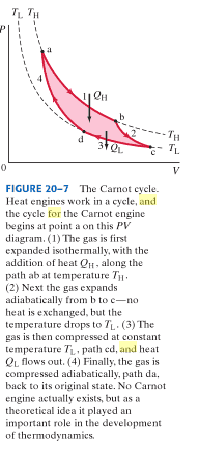# Volumes at C and D in a Carnot cycle

## Homework Statement

A Carnot cycle, shown in Fig. 20-7, has the following conditions: Va = 7.5 L, Vb = 15 L, TH = 470°C, and TL = 260°C. The gas used in the cycle is 0.50 mil of a diatomic gas, y = 1.4. Calculate (a) the pressures at a and b; (b) the volumes at c and d. (The rest of the problem...)

PVy = Constant

## The Attempt at a Solution

I already solved part (a), with Pa = 4.1 x 105 Pa and Pb = 2.1 x 105 Pa.

For part (b), I tried
PVy = constant
nRT⋅Vy-1 = constant.

Annnnd then I get lost. I don't understand what constant represents or if I am even on the right track. The book says Vc = 34 L and Vd = 17 L.

vela
Staff Emeritus
Homework Helper
What do the points a, b, c, and d represent in the Carnot cycle? You didn't post the figure.

My apologies, here is the figure:Points a, b, c, and d represent stages of the Carnot engine as it works.

vela
Staff Emeritus